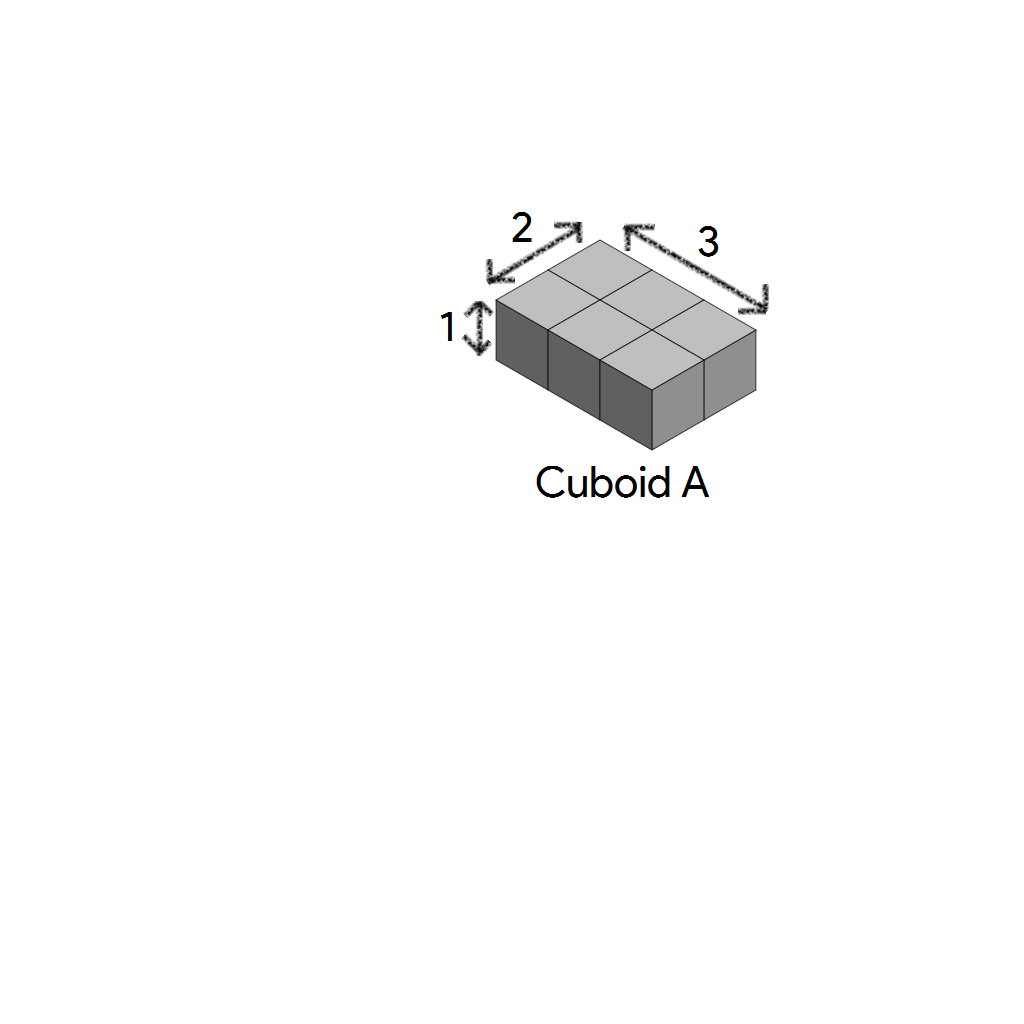# Turning Cuboids Into A Cube

Geometry Level 3$N$ cuboid $A$'s are arranged to form the smallest cube possible.

Find the total surface area of the cube.

×